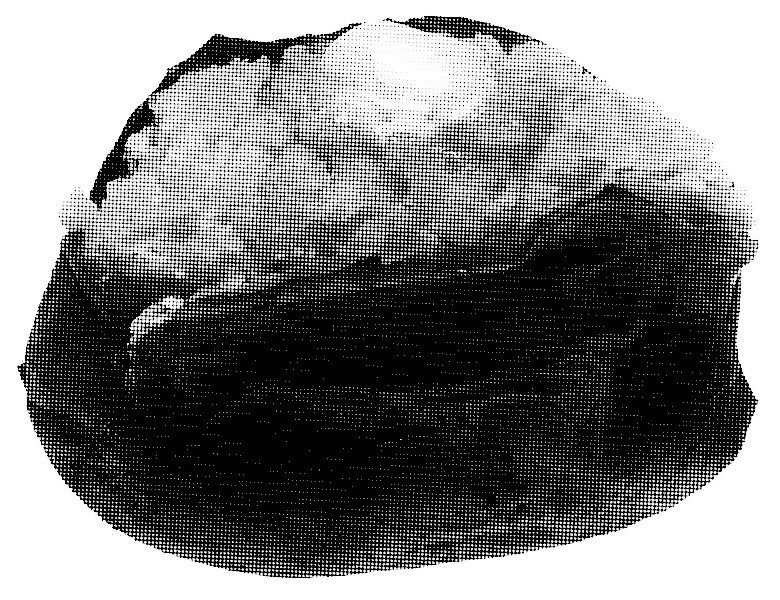# Page 3 model: The potato Earth

How Kelvin estimated the age of the Earth by thinking about a baked potatoWilliam Thomson—better known as Lord Kelvin—was a British mathematician who became interested in the temperature of the Earth around the late 19th century. Global temperatures have risen since his time, but our ability to predict the future of our climate has not, despite the enormous threat global warming poses to our planet.One of these is Earth…

Much like our current ignorance of the future, the 19th century was relatively ignorant of the past: Kelvin’s interest in global temperature was for a method to determine the age of the Earth.

His idea was roughly as follows: assume the Earth is like a baked potato left out on the kitchen table. While the skin has cooled completely, the hot interior mash is just waiting to scald the mouth of an unsuspecting potato thief. Over time, the parts of the potato near the surface will cool down more quickly, so the rate of cooling is proportional to the change in temperature gradient through the potato:$$\frac{\partial T}{\partial t} = \kappa_p \frac{\partial^2 T}{\partial x^2},$$where temperature $T(x,t)$ depends on time $t$ and distance to the surface $x$, and $\kappa_p$ is a constant depending upon the potato.

By assuming the skin is perfectly cool:$$T(0,t) = 0,$$and assuming a perfectly baked potato, ie the initial temperature is uniform:$$\frac{\partial T}{\partial x}=0 \quad \text{everywhere at t=0,}$$Kelvin found an approximate solution to this equation, and was able to estimate the age of the Earth to around a million years. Of course, this is out by a factor of about a million, but was more accurate than many contemporary estimates.…the other is a baked potato

It is a common misconception that this model gives inaccurate estimates because Kelvin was unaware of the decay of radioactive materials throughout the Earth’s crust, but these effects are relatively minor.

A more sensible correction has to do with thermal convection currents in the Earth’s mantle, but anyone who thinks either of these happen in a potato is clearly baked.Jakob is a PhD student and mathematician from London and works mainly in differential geometry. In his spare time, he likes to draw, and think about mathematics in art.